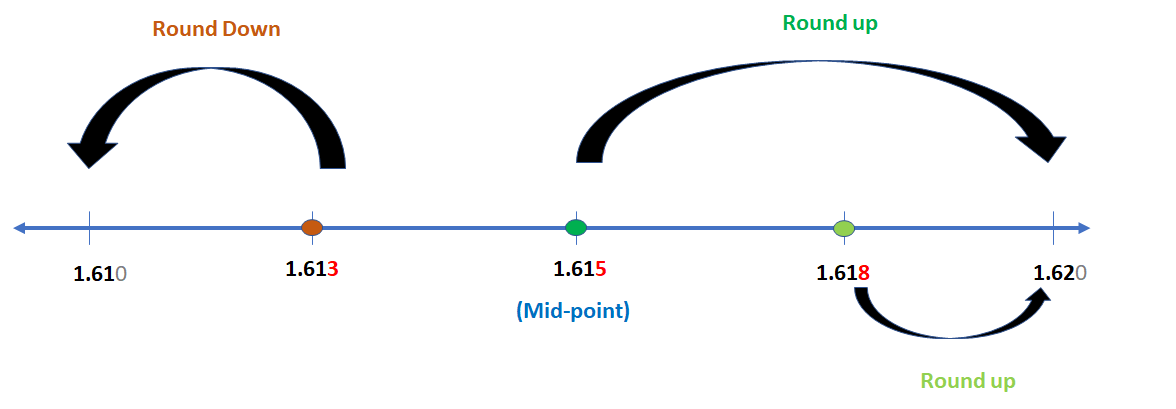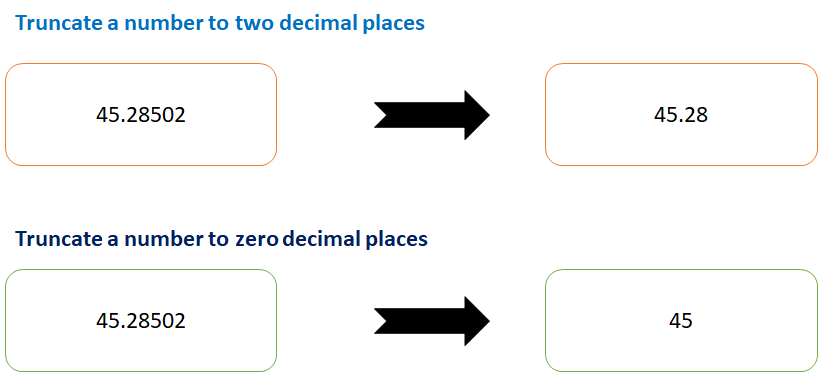Select Page

# Truncate, Round Down and Round Up Decimal Number in SAP ABAP

by | Jun 3, 2018 | ABAP Programs

Home » SAP » ABAP » ABAP Programs » Truncate, Round Down and Round Up Decimal Number in SAP ABAP

Preface – This post is part of the ABAP Programs series.

Many times in SAP ABAP, there is a requirement to Truncate, Round Down and Round Up a Decimal Number. In that case, we may use the predefined ABAP keywords to achieve the same. In this article, we will be discussing them altogether.

## Introduction

We can truncate (remove decimal values), round down (5.5 to 6) and round up (5.5 to 5) in ABAP. To achieve these functionalities, ABAP has provided keywords like FLOOR, CEIL and TRUNC. These keywords are implemented in given ABAP program:Round Down and Round Up Decimal Number in SAP ABAP – Image IllustrationTruncate, Round Down and Round Up Decimal Number in SAP ABAP – Image Illustration

## ABAP Program

```Data: tp_deci3 type p decimals 3,
tp_deci2 type p decimals 2.
tp_deci3 = '123.456'.
tp_deci2 = ( floor( tp_deci3 * 100 ) ) / 100. "always rounded down
Write tp_deci2.
tp_deci2 = ( ceil( tp_deci3 * 100 ) ) / 100. "Always rounded up
Write tp_deci2.
tp_deci2 = ( trunc( tp_deci3 * 100 ) ) / 100. "Truncate a number
Write tp_deci2.
```

## Explanation

In the program, mentioned above, we have implemented following, step by step:

1. Initially, we have defined two variables: tp_deci3 and tp_deci2. Both of these variables are of type i.e. packed (like float, it can have decimal values too).  tp_deci3 can have 3 decimal places while  tp_deci2 can have 2 decimal places.
2. Now, we have simply implemented the ABAP keywords: FLOOR, CEIL and TRUNC; one by one. [How it works has been illustrated via images, above].
3. Later, we have printed the result as output.

## Author

•A Full Stack Developer with 10+ years of experience in technical content creation.

•